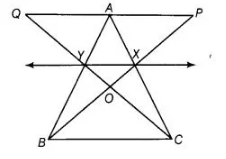# In figure X and Y are the mid-points of AC`
Question:

In figure X and Y are the mid-points of AC and AB respectively, QP || BC and CYQ and BXP are straight lines. Prove that ar (ΔABP) = ar (ΔACQ).Thinking Process

1. Firstly, use the theorem that joining the mid-points of the two sides of a triangle is parallel to the third side.
2. Further, use the theorem that triangles on the same base and between the same
parallels are equal in area. Use this theorem by considering different triangles and prove the required result

Solution:

Given X and Y are the mid-points of AC and AB respectively. Also, QP|| BC and CYQ, BXP are straight lines.

To prove ar (ΔABP) = ar (ΔACQ)

Proof Since, X and Y are the mid-points”of AC and AB respectively.

So, XY || BC

We know that, triangles on the same base and between the same parallels are equal in area. Here, ΔBYC and ΔBXC lie on same base BC and

between the same parallels BC and XY.

So, ar (ΔBYC) = ar (ΔBXC)

On subtracting ar (ΔBOC)from both sides, we get

ar (ΔBYC) – ar (ΔBOC) = ar (ΔBXC) – ar (ΔBOC)

=» ar (ΔBOY) = ar (ΔCOX)

On adding ar (ΔXOY) both sides, we get

ar (ΔSOY) + ar (ΔXOY) = ar (ΔCOX) + ar (ΔXOY)

=> ar (ΔBYX) = ar (ΔCXY) …(i)

Hence, we observe that quadrilaterals XYAP and YXAQ are on the same base XY and between the same parallels XY and PQ.

ar (XYAP) = ar (YXAQ) …(ii)

On adding Eqs. (i) and (ii), we get

ar (ΔBYX) + ar (XYAP) = ar (ΔCXY) + ar (YXAQ)

=> ar (ΔABP) = ar (ΔACQ) Hence proved.